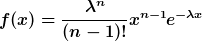# What is Erlang

## Erlang distribution

continuous probability distribution. A continuous random variable X is called Erlang-distributed with the parameters n and λ if it is the density functionfor x> 0 has; n is a natural number and λ is a positive number. The associated expected value is n / λ and the variance is n / λ2. A sum of n stochastically independent random variables, each of which has an exponential distribution with an identical parameter λ, is Erlang-distributed with the parameters n and λ. The Erlang distribution therefore plays a role in practice in connection with the statistical analysis of lifetimes and residence times. An Erlang distribution is a special gamma distribution.